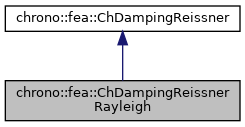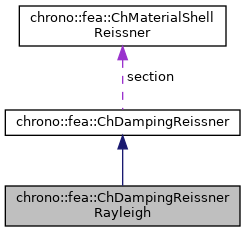chrono::fea::ChDampingReissnerRayleigh Class Reference

## Description

Simple Rayleight damping of a Reissner-mindlin shell, where damping is proportional to stiffness via a beta coefficient.

In order to generalize it also in case of nonlinearity, the full element tangent stiffness matrix cannot be used (it may contain negative eigenvalues) and it can't be used to recover instant nodal caused by damping as F=beta*K*q_dt so it is generalized to the following implementation at the material stress level

  {n,m}=beta*[E]*{e',k'}


where

• beta is the 2nd Rayleigh damping parameter
• [E] is the 6x6 shell stiffness matrix at the undeformed unstressed case (hence assumed constant)
• {e',k'} is the speed of deformation/curvature Note that the alpha mass-proportional parameter (the first of the alpha,beta parameters of the original Rayleigh model) is not supported.

#include <ChMaterialShellReissner.h>

Inheritance diagram for chrono::fea::ChDampingReissnerRayleigh:[legend]
Collaboration diagram for chrono::fea::ChDampingReissnerRayleigh:[legend]

## Public Member Functions

ChDampingReissnerRayleigh (std::shared_ptr< ChElasticityReissner > melasticity, const double &mbeta=0)
Construct the Rayleigh damping model from the stiffness model used by the shell layer. More...

virtual void ComputeStress (ChVector<> &n_u, ChVector<> &n_v, ChVector<> &m_u, ChVector<> &m_v, const ChVector<> &deps_u, const ChVector<> &deps_v, const ChVector<> &dkur_u, const ChVector<> &dkur_v, const double z_inf, const double z_sup, const double angle)
Compute the generalized cut force and cut torque, caused by structural damping, given actual deformation speed and curvature speed. More...

virtual void ComputeDampingMatrix (ChMatrixRef R, const ChVector<> &deps_u, const ChVector<> &deps_v, const ChVector<> &dkur_u, const ChVector<> &dkur_v, const double z_inf, const double z_sup, const double angle)
Compute the 6x6 tangent material damping matrix, ie the jacobian [Rm]=dstress/dstrainspeed. More...

double GetBeta ()
Get the beta Rayleigh parameter (stiffness proportional damping)

void SetBeta (const double mbeta)
Set the beta Rayleigh parameter (stiffness proportional damping)Public Attributes inherited from chrono::fea::ChDampingReissner
ChMaterialShellReissnersection

## ◆ ChDampingReissnerRayleigh()

 chrono::fea::ChDampingReissnerRayleigh::ChDampingReissnerRayleigh ( std::shared_ptr< ChElasticityReissner > melasticity, const double & mbeta = 0 )

Construct the Rayleigh damping model from the stiffness model used by the shell layer.

This is important because the Rayleigh damping is proportional to the stiffness, so the model must know which is the stiffness matrix of the material. Note: melasticity must be alreay set with proper values: its [E] stiffness matrix will be fetched just once for all.

## ◆ ComputeDampingMatrix()

 void chrono::fea::ChDampingReissnerRayleigh::ComputeDampingMatrix ( ChMatrixRef R, const ChVector<> & deps_u, const ChVector<> & deps_v, const ChVector<> & dkur_u, const ChVector<> & dkur_v, const double z_inf, const double z_sup, const double angle )
virtual

Compute the 6x6 tangent material damping matrix, ie the jacobian [Rm]=dstress/dstrainspeed.

In this model, it is beta*[E] where [E] is the 12x12 stiffness matrix at material level, assumed constant

Parameters
 R 12x12 material damping matrix values here deps_u time derivative of strains along u direction deps_v time derivative of strains along v direction dkur_u time derivative of curvature along u direction dkur_v time derivative of curvature along v direction z_inf layer lower z value (along thickness coord) z_sup layer upper z value (along thickness coord) angle layer angle respect to x (if needed) -not used in this, isotropic

Reimplemented from chrono::fea::ChDampingReissner.

## ◆ ComputeStress()

 void chrono::fea::ChDampingReissnerRayleigh::ComputeStress ( ChVector<> & n_u, ChVector<> & n_v, ChVector<> & m_u, ChVector<> & m_v, const ChVector<> & deps_u, const ChVector<> & deps_v, const ChVector<> & dkur_u, const ChVector<> & dkur_v, const double z_inf, const double z_sup, const double angle )
virtual

Compute the generalized cut force and cut torque, caused by structural damping, given actual deformation speed and curvature speed.

Parameters
 n_u forces along u direction (per unit length) n_v forces along v direction (per unit length) m_u torques along u direction (per unit length) m_v torques along v direction (per unit length) deps_u time derivative of strains along u direction deps_v time derivative of strains along v direction dkur_u time derivative of curvature along u direction dkur_v time derivative of curvature along v direction z_inf layer lower z value (along thickness coord) z_sup layer upper z value (along thickness coord) angle layer angle respect to x (if needed)

Implements chrono::fea::ChDampingReissner.

The documentation for this class was generated from the following files:
• /builds/uwsbel/chrono/src/chrono/fea/ChMaterialShellReissner.h
• /builds/uwsbel/chrono/src/chrono/fea/ChMaterialShellReissner.cpp